Motion under gravity

Start this free course now. Just create an account and sign in. Enrol and complete the course for a free statement of participation or digital badge if available.

Free course

# 1: Gravitational force

The gravitational force of attraction exists between objects. This force is the reason you feel yourself pulled towards the Earth.

## Activity 1

How does the magnitude of the gravitational force between two objects change when the masses of the objects increase, and when their separation increases?

### Discussion

The magnitude of the gravitational force increases when the masses of the objects increase, and it decreases as their separation increases.

The gravitational force that you experience depends on the mass of the Earth, and on the separation between you and the mass of the Earth. For a large (approximately) spherical object like the Earth, it is the separation between you and the centre of the Earth that is relevant, and this separation is essentially the same for all objects close to the Earth's surface.

## Activity 2

Does this mean that every object on the Earth's surface experiences the same gravitational force?

### Discussion

No! The force experienced also depends on the mass of the object.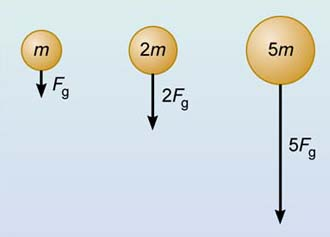Figure 1 Gravitational force is proportional to mass.

In fact, the gravitational force F g experienced by an object is proportional to its mass m (Figure 1), so we can write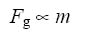Thus the force of gravity you experience due to the Earth is proportional to your own mass. Someone with a mass of 50 kg experiences only half the gravitational force felt by someone with a mass of 100 kg.

## Activity 3

How can we express this relationship between gravitational force and mass as an algebraic equation?

### Discussion

We can change a proportionality relationship between two quantities into an equation by introducing a constant of proportionality.

In this case, the equation linking the gravitational force F g with the mass m that experiences the force can be written as: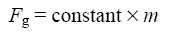To discover the meaning of the constant in this equation, let's consider the motion of an object of mass m that is falling towards the Earth, for example, an apple falling from a tree. Newton's second law of motion states that the acceleration a of the object is related to the unbalanced force F acting on it by the equation: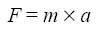We will assume that the force due to air resistance is very small, and can be neglected, so that the object falls freely, acted on by only the gravitational force F g. So the unbalanced force F in Equation 2 must be F g. Therefore we can rewrite Newton's second law for a freely-falling object as: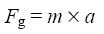We now have two equations for F g, 1 and 3, and so we can equate the right-hand sides as follows: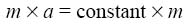If we divide both sides of this equation by m, this leads to an important conclusion about the acceleration a of an object acted on by the force of gravity alone: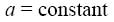The acceleration due to gravity experienced by any object that is falling freely close to the Earth's surface is a constant. It has the same value, irrespective of the mass of the object.

This was the surprising result discovered about 400 years ago by Galileo, who is alleged to have dropped two cannonballs of different mass from the top of the Leaning Tower of Pisa and observed that they took approximately the same time to reach the ground (Figure 2).Figure 2 Galileo's experiment, showing that cannonballs with different mass are accelerated equally by gravity. Historians doubt that Galileo performed this experiment, though he certainly did propose that gravity pulls large and small objects towards the Earth with the same acceleration. His proposal was so contentious at the time that he was forced to leave the University of Pisa.

The value of the acceleration due to gravity is always represented by the symbol g. It actually increases by about 0.5% between the Equator and the poles, and there are two reasons for this; one is the slightly non-spherical shape of the Earth and the other is the Earth's rotation. However, for the purpose of this course, we will usually ignore this small variation and will take the value of the acceleration due to gravity near to the Earth's surface to be 9.8 m s−2.

## Question 1

What is the force due to gravity acting on (a) a person of mass 80 kg, and (b) a 100 g bar of chocolate?

We know that when any object near the Earth's surface is acted on by the gravitational force alone, it has acceleration g=9.8 m s−2. So applying Newton's second law of motion, the gravitational force is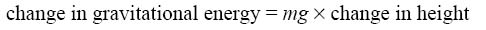1. For a person with m= 80 kg: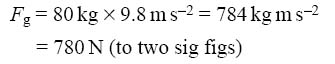2. For a 100 g bar of chocolate, we substitute m=0.10 kg into the equation F g=mg, and in this case the gravitational force is 0.98 N, which is about 1 N. {So if you want to know what 1 N feels like, pick up a 100 g object and experience the force that it exerts on your hand.}

The gravitational force F g experienced by an object is called the weight of the object. Now we showed that when an object is acted on by the force of gravity F g alone, then its acceleration is g, the acceleration due to gravity. So according to Newton's second law: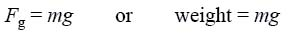Note that, whereas the mass m of an object is constant (provided it does not lose or gain matter), its weight F g is the gravitational force it experiences — and this depends on where on Earth (Figure 4.3) or in the Universe the object is located.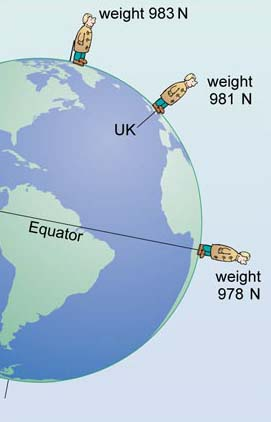Figure 3 The small variation of the acceleration due to gravity with latitude means that the weight of a 100 kg person depends on their location on Earth. At all three locations the person's weight is 980 N (and g=9.8 m s−2) to two significant figures.

## Question 2

What is the weight of a person with a mass of 80 kg (a) on the Earth (assuming g=9.8 m s−2), and (b) on the Moon where the acceleration due to gravity is roughly 1.6 ms−2?

(a) A person with mass m has weight mg. On Earth, g=9.8 m s−2, so the weight of an 80 kg person is 80 kg×9.8 ms−2=780 N (to two sig figs). {This is the same as the value you calculated in Question 1, since the weight of an object is the same as the gravitational force that acts on it. Next time you are asked your weight, you might like to give the value in newtons - and then explain to your friends the difference between the scientific and everyday use of the term weight!}

(b) On the Moon, g=1.6 m s−2, so in this case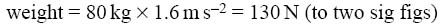{This shows that the weight of an object on the Moon is roughly one-sixth of its weight on Earth, even though its mass is the same in both places.}

S103_3